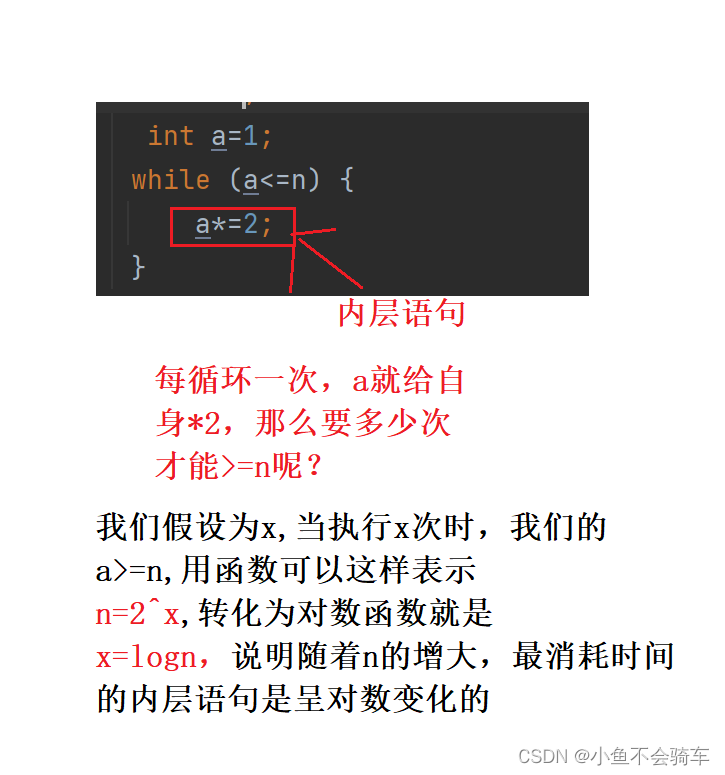# 如果各位同学还对时间复杂度有疑问？看这一篇就可以啦！

🎇🎇🎇作者：
@小鱼不会骑车
🎆🎆🎆专栏：
《java练级之旅》
🎓🎓🎓个人简介：• 时间复杂度
• 前言
• 时间复杂的讲解
• 如何简化时间复杂度
• 时间复杂度的计算

# 时间复杂度

## 前言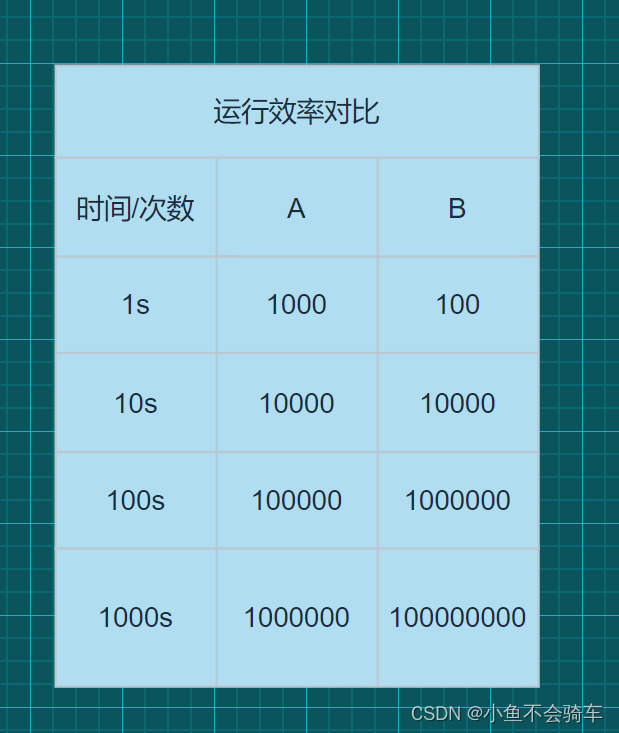## 时间复杂的讲解

``````   public static void main(String[] args) {
int n=100;
while ((n)!=0) {
n--;
}
}
``````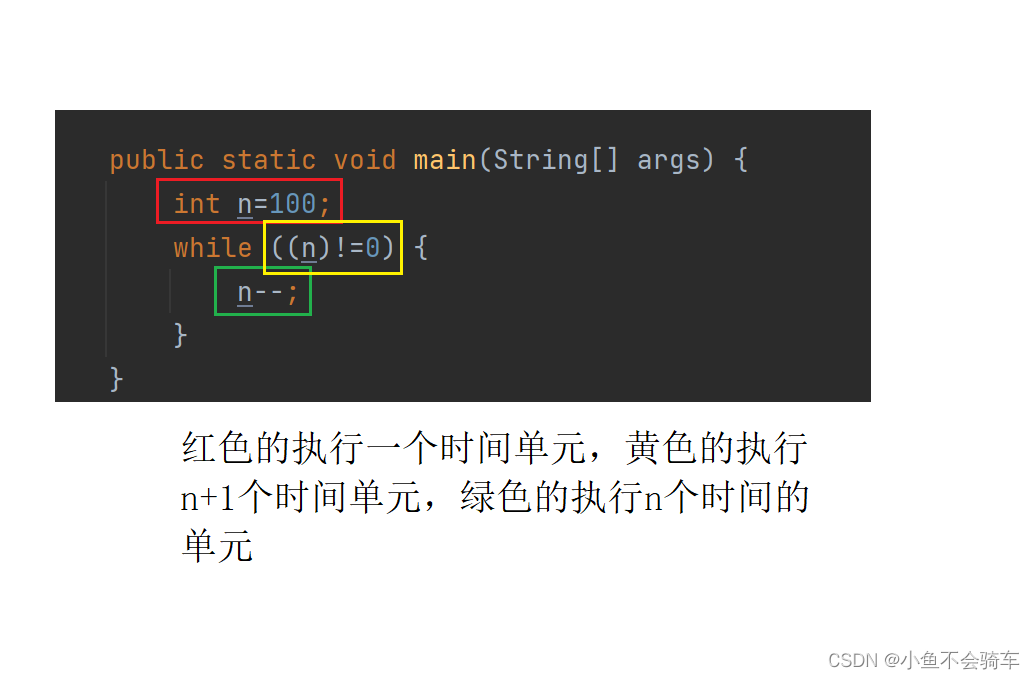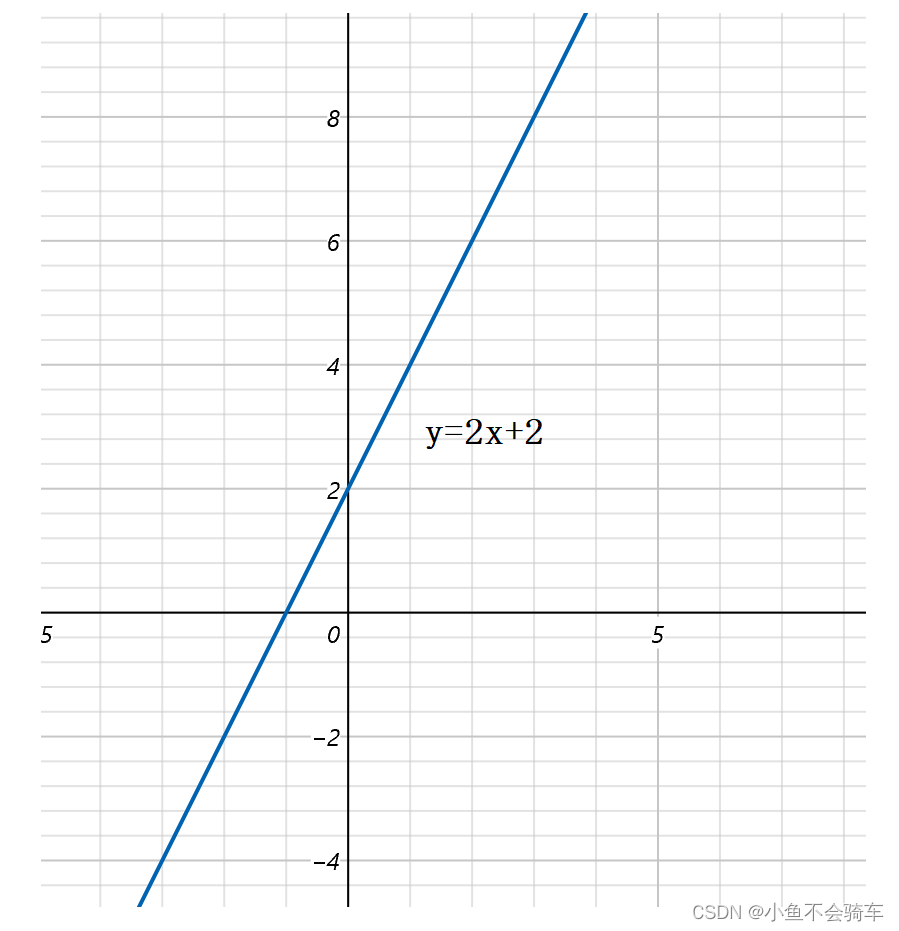## 如何简化时间复杂度T(n)=n+1 忽略常数项 T(n)~n

T(n)=n+n^2 忽略低阶项 T(n)~n^2

T(n)=3n 忽略最高阶的系数 T(n)~n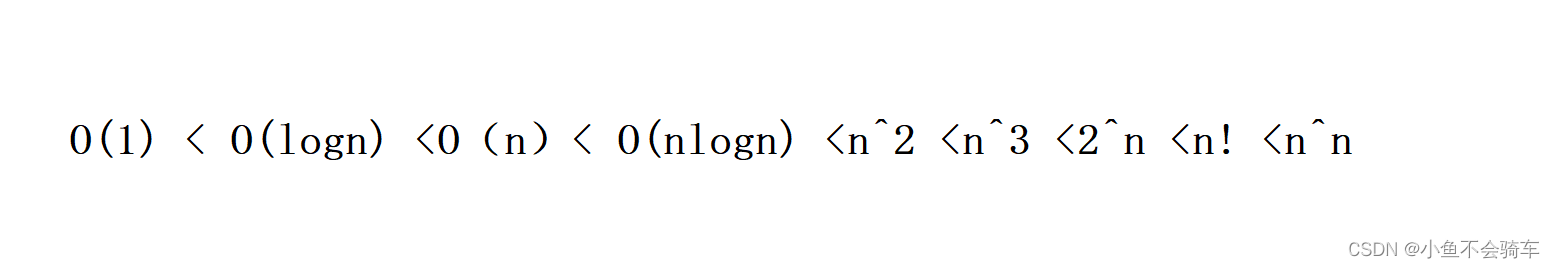## 时间复杂度的计算

1.用常数1取代运行时间中所有加法常数
2.修改后的函数只保留最高阶项
3.如果最高阶项存在，并且不是1，则忽略这个最高项数的系数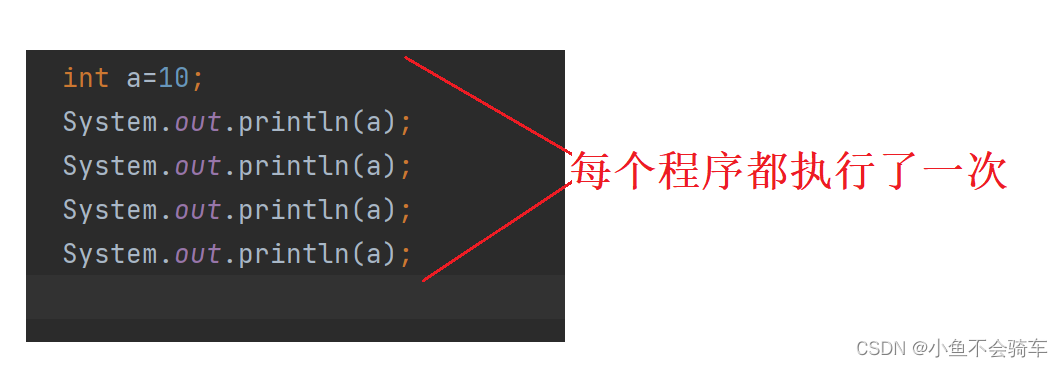O(1)也被称为常数阶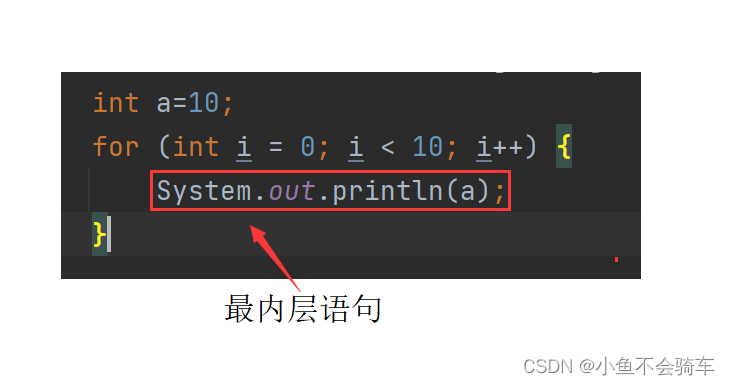.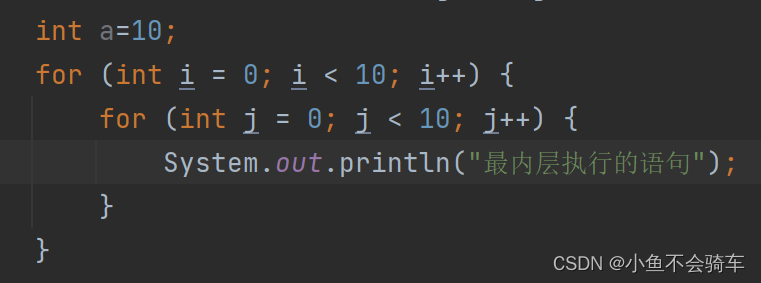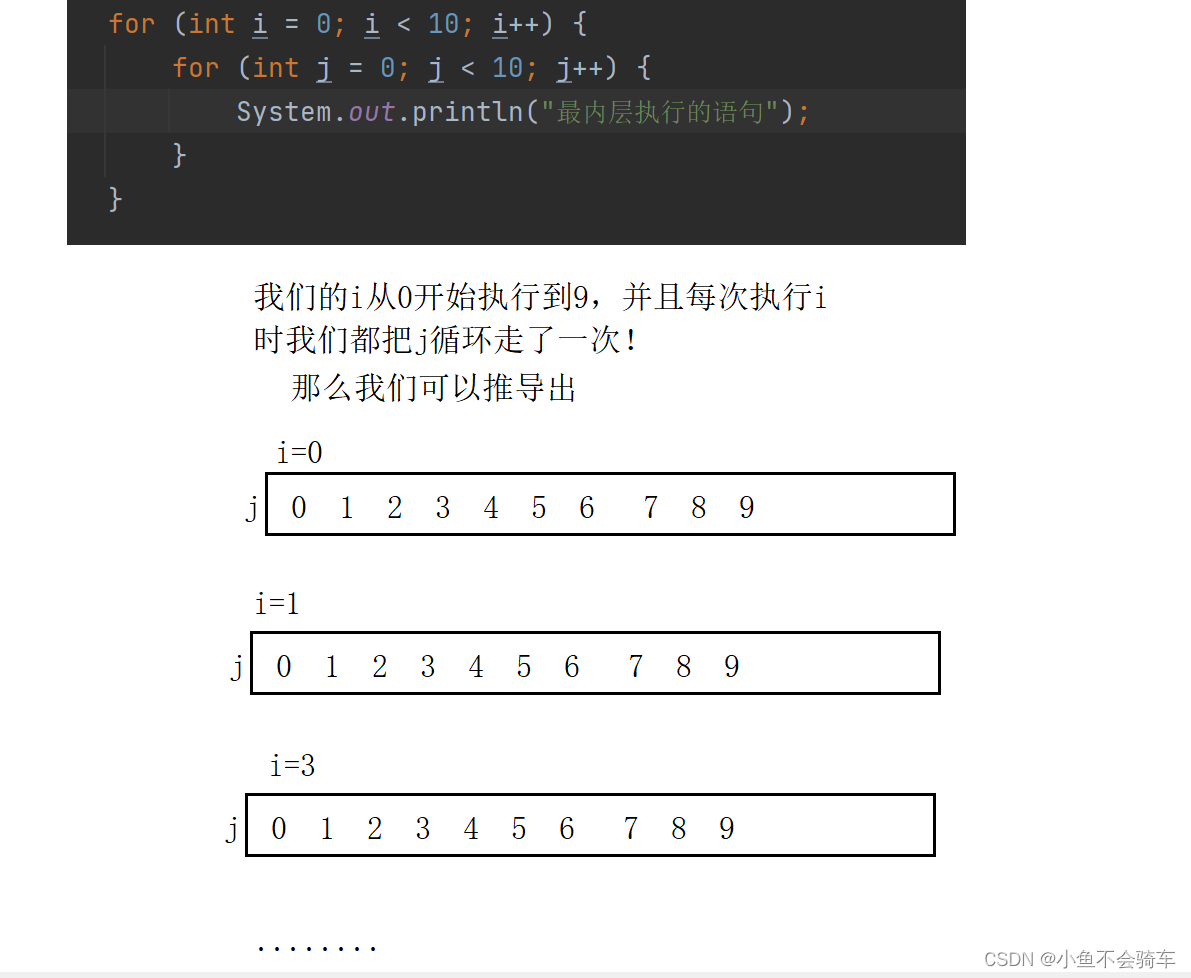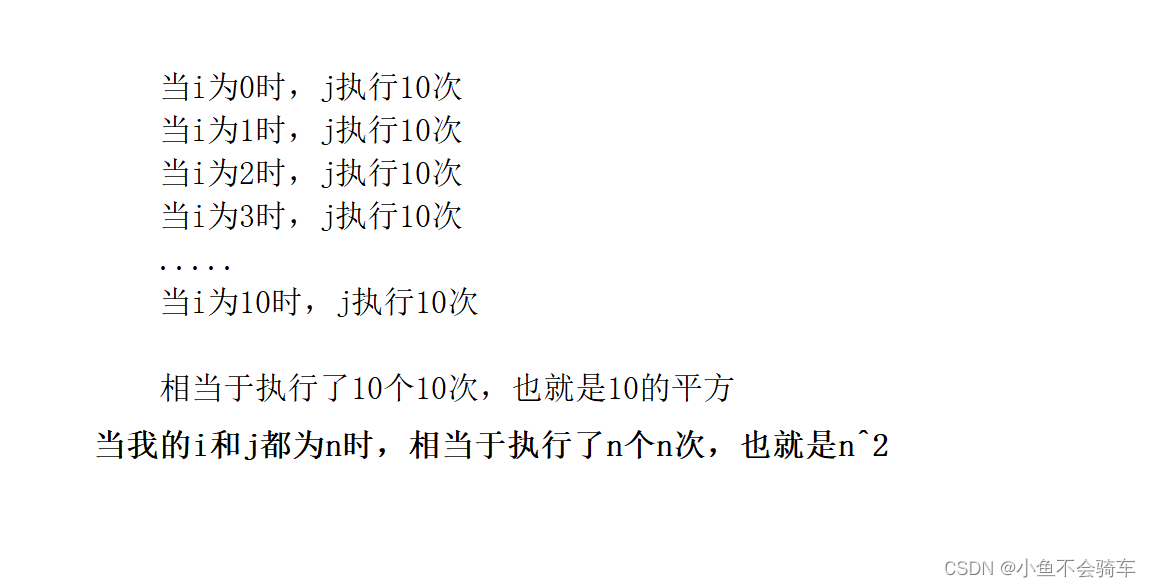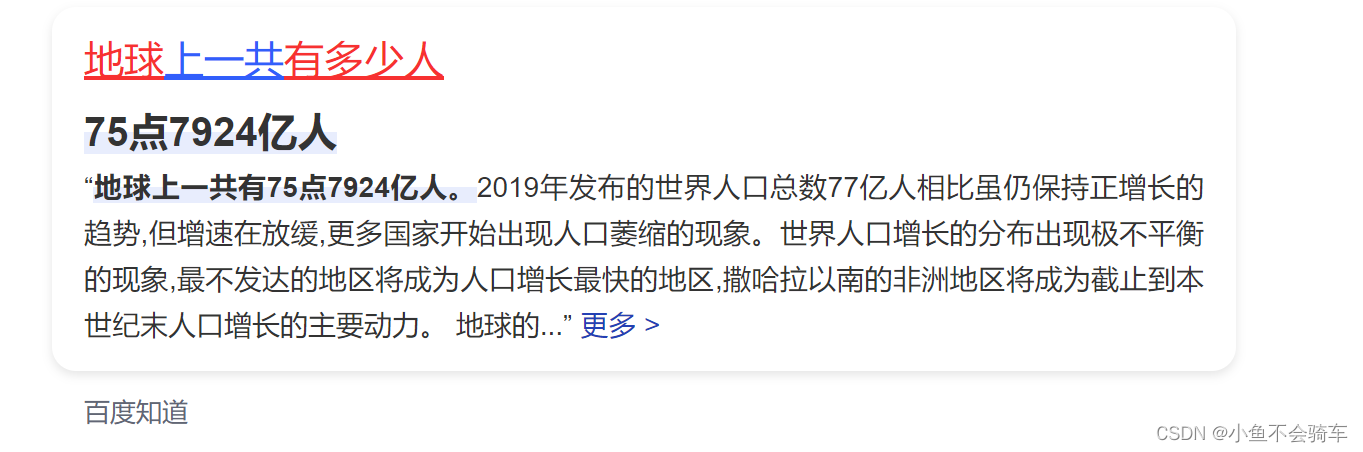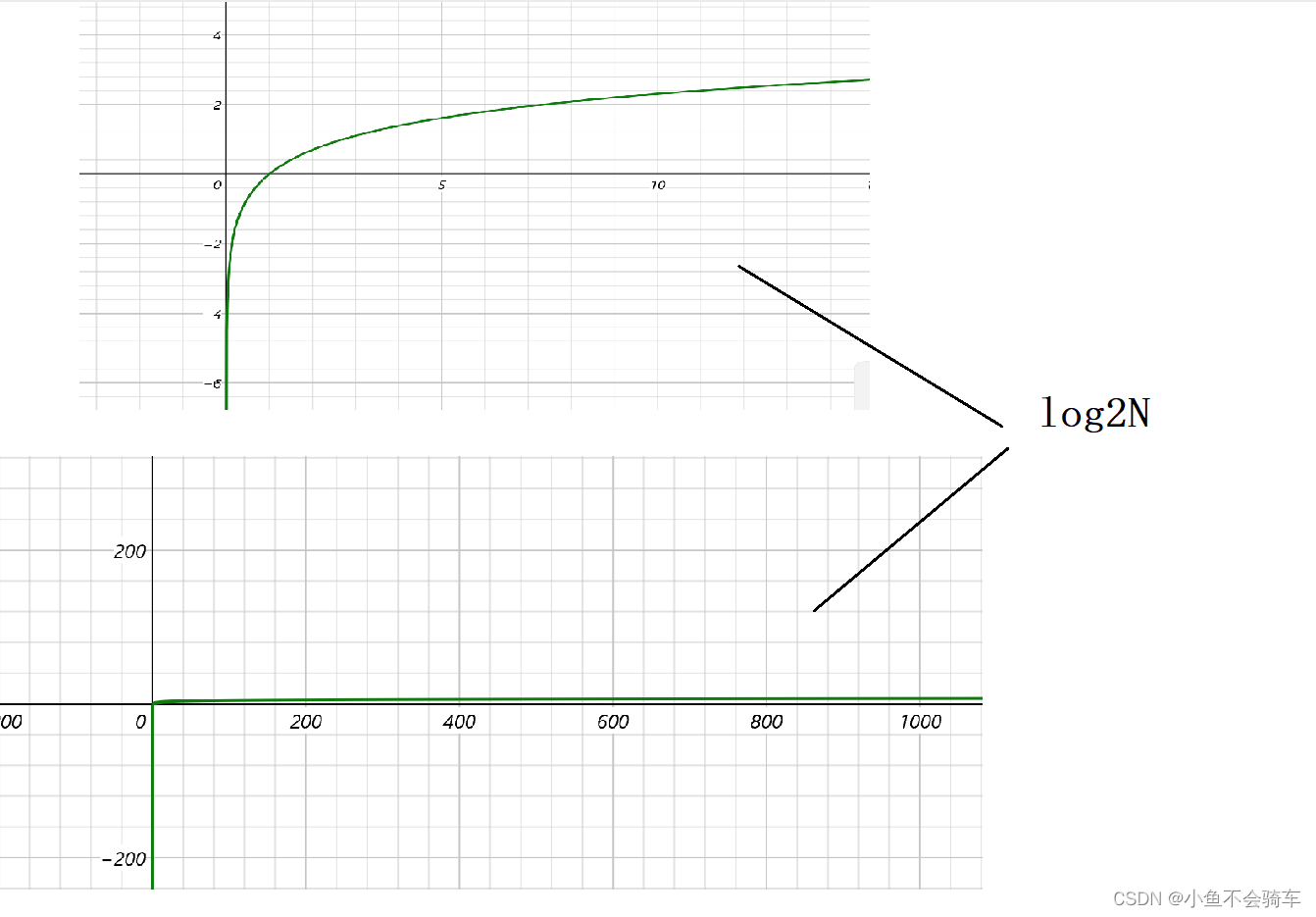``````	int a=1;
while (a<=n) {
a*=2;
}
``````